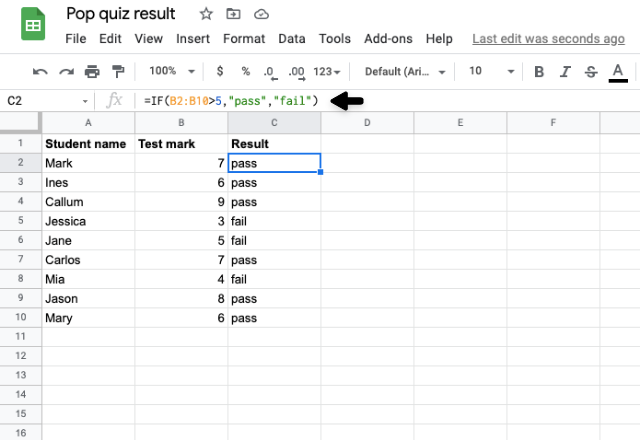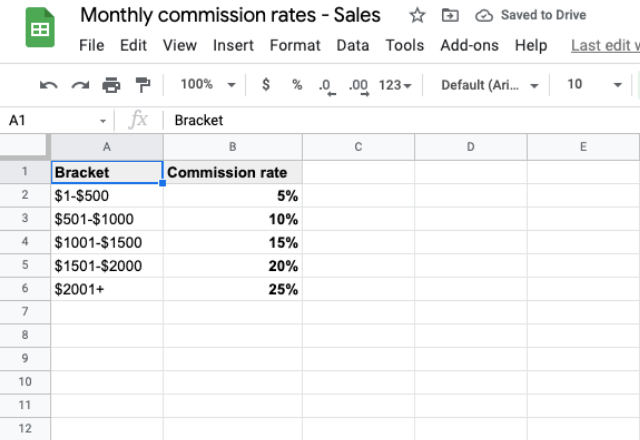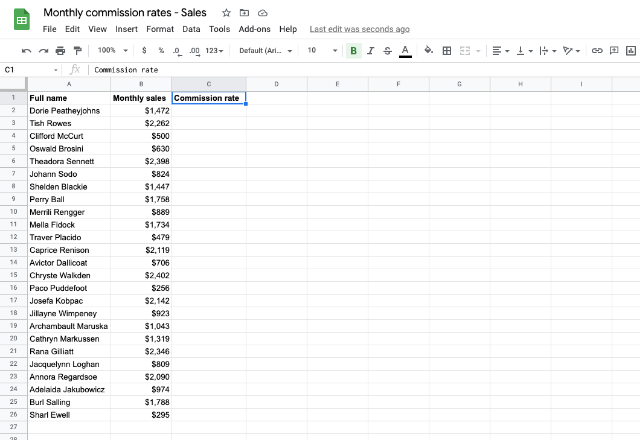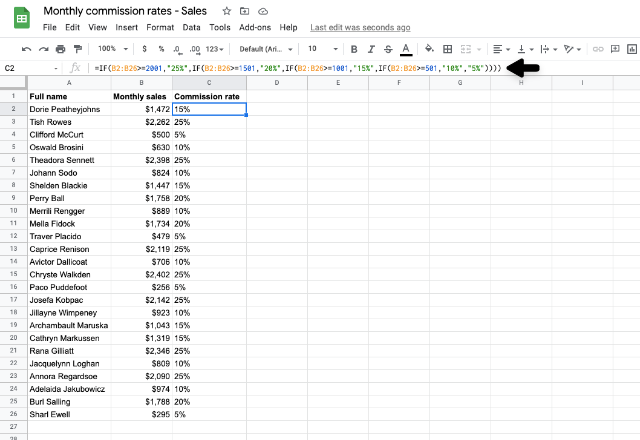# How to use the nested IF function in ExcelWritten by
Holly Meikle

Excel has many intelligent functions that are extremely useful in sorting and analyzing large amounts of data in a spreadsheet. The nested IF function in Excel is one of the more advanced functions you can use. It allows you to test multiple conditions of your data to identify or categorize it automatically.

The nested IF function can be handy in various situations because of its ability to test conditions. There are many circumstances in which we have to assign values to data based on pre-existing categories. For example, let’s say you need to calculate the commission for each of your team members’ sales in the last month. Using the nested IF function, you can input the commission rate categories and automatically assign a rate to each member in just a matter of seconds.

Although this is a more technical formula to use in Excel, it can save you a lot of time. This article will offer a complete breakdown of how to use the nested IF function in Excel, with some useful examples that you can implement in your operations.

### The IF function in Excel

The IF function in Excel is a logical function. Using the standard IF formula, you can test a condition. If the condition is met, one value is returned. If the condition is not met, another value is returned. Often, this is a simple ‘true’ or ‘false’.

The following is the formula for the IF function:

=​​IF([condition], [value_if_true], [value_if_false])

For example, let’s say you want to know whether your students have passed the latest pop quiz. If they receive over 5 marks, they have passed. Anything below, they have failed.

The formula we would use is:

=IF(B2:B10>5,”pass”,”fail”)With the IF function, you can only use one condition. So what if you have multiple conditions you want to test on data? That’s when we use the nested IF function.

### What is the nested IF function?

The nested If function works in exactly the same way as the If function. However, this allows you to test multiple conditions at once. If a data value doesn’t meet the first condition, it may meet the second. If it doesn’t meet the second condition, it may meet the third, etc. You can enter up to 64 different conditions in a single nested IF formula (however, this is not advisable).

#### How to nest an IF function

Use the following formula as the basis for your nested IF function:

=IF([condition1],[value_if_true1], IF([condition2],[value_if_true2],[value_if_false]))

You can continue to add as many conditions as you like to your formulas. Just remember:

• Make sure that the [value_if_false] is at the end of the nested IF formula
• Include the same number of closed brackets ‘)’ as there are conditions at the end of the formula (5 conditions = 5 brackets)

Now that you have the syntax for the nested IF formula, let’s put it into practice.

### How to use the nested IF function in Excel

Let’s take our original example to learn how to use the nested IF function in excel. We want to calculate the commission rates for each of your sales team members regarding their sales in the last month. Here are the commission rates:These commission rate brackets will be our categories. We can create multiple conditions that test these categories against each sales value using the nested IF function. As a result, Excel assigns the correct commission rate to each value.

Here is our set of data to apply the commission rates with our nested IF statement:To successfully assign the right commission rate to each sales member, the nested IF formula would look like this:

=IF(B2:B26>2001, 25%, IF(B>1501,20%, IF(B>1001,15%, IF(B>501,10%, 5%))))

In our condition, we are testing if our data is more than (>) a specific value. Because of this, we use the starting value of each bracket (the lowest value within each category).

It’s also important to note that we have started the formula with the highest commission rate bracket. If we started at the lowest one, nearly all the data would test the condition as “true”. So, always remember to create your nested IF formulas starting with the most difficult condition to pass, then follow in descending order.

Using this function, we receive the following results:As you can see, the nested IF function has correctly assigned the commission rate to each sales member.

### When to use the nested IF function

Although the nested IF function is a fantastic way to quickly identify and categorize your data, it can get very complicated. We recommend using the nested IF function to test clear categories that aren’t too numerous. If you find yourself in a situation where this is not possible, take a look at alternative Excel functions such as VLOOKUP. You can learn more about this in our blog post on how to use the VLOOKUP Excel function.

Alternatively, if you are struggling with entering the function correctly (missing out brackets or forgetting to add the IF statement to every new condition), you may want to look into Excel’s newer IFS function . This works in the same way as the standard nesting IF function; it just simplifies the formula input.

### Conclusion

And there we have it! The nested IF function in Excel is a fantastic formula to use to categorize and manage large amounts of data in a short amount of time. Although this function is more advanced than others, it’s applicable in many situations, so it is worth mastering. If you would like to explore other valuable functions available in Excel, discover our related articles below!